# Images Formed by Spherical Mirrors

## Introduction

• Drawing the ray diagrams is an ideal way to illustrate the formation of images by spherical mirrors.

• The intersection of at least two reflected rays give the correct position of image of the point object.

• The following table illustrates the image formed by a concave mirror for different positions of the given object −

Position of Object Position of Image Size of Image Nature of Image Image
At infinity At the focus F Highly diminished, pointsized Real and inverted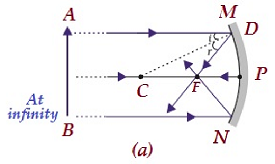Beyond C B/w F and C Diminished Real and inverted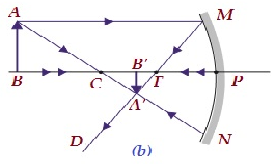At C At C Same size Real and inverted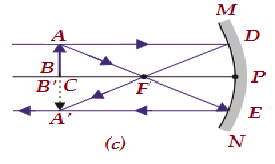B/w C and F Beyond C Enlarged Real and inverted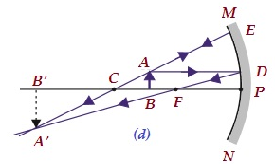At F At infinity Highly enlarge Real and inverted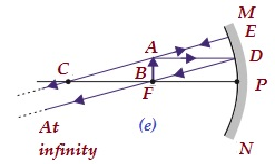B/w P and F Behind the mirror Enlarged Virtual and erect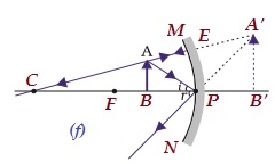## Uses of Concave Mirror

• In order to get powerful parallel beams of light, concave mirrors are universally used in torches, search-lights, and vehicles headlights.

• Concave mirror is also used in barber’s saloon, as it gives larger view.

• Concave mirror is also used by dentists, to see the large images of the teeth of patients.

• Large concave mirrors are used to concentrate sunlight to produce maximum heat in the solar furnaces.

## Image formation by a Convex Mirror

• The following table illustrates the image formed by a concave mirror for different positions of the given object −

Position of Object Position of Image Size of Image Nature of Image Image
At infinity At the focus F, behind the mirror Highly diminishe d, point sized Virtual and erect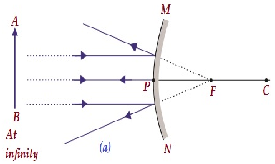B/w infinity and pole of the mirror B/w P and F, behind the mirror Diminishe d Virtual and erect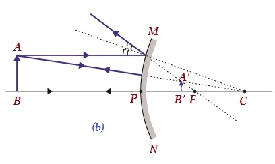## Uses of Convex Mirrors

• In all vehicles, convex mirrors are universally used as rear-view (wing) mirrors.

• In vehicles, convex mirrors are preferred, as they give though diminished, but an erect image.

### Mirror Formula

• The formula is expressed as:

• $$\frac{1}{v} + \frac{1}{u} = \frac{1}{f}$$

• Mirror formula expresses the relationships among the object-distance (i.e. u), image-distance (i.e. v), and focal length (i.e. f) of a spherical mirror.